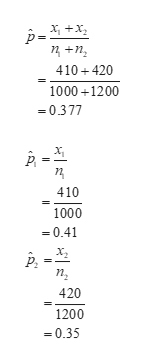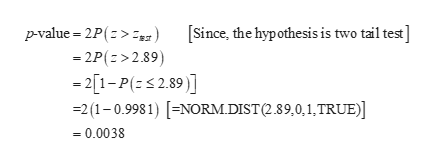Question
2 views
A certain financial services company uses surveys of adults age 18 and older to determine if personal financial fitness is changing over time. A recent sample of 1,000 adults showed 410 indicating that their financial security was more than fair. Suppose that just a year before, a sample of 1,200 adults showed 420 indicating that their financial security was more than fair.
(a)
State the hypotheses that can be used to test for a significant difference between the population proportions for the two years. (Let p1 = population proportion most recently saying financial security more than fair and p2 = population proportion from the year before saying financial security more than fair. Enter != for ≠ as needed.)
H0:
p1​−p2​=0

Ha:
p1​−p2​!=0

(b)
Conduct the hypothesis test and compute the p-value. At a 0.05 level of significance, what is your conclusion?
Find the value of the test statistic. (Use
p1 − p2.

p-value =
Reject H0. There is sufficient evidence to conclude the population proportions are not equal. The data suggest that there has been a change in the population proportion saying that their financial security is more than fair.Do not reject H0. There is insufficient evidence to conclude the population proportions are not equal. The data do not suggest that there has been a change in the population proportion saying that their financial security is more than fair.     Reject H0. There is insufficient evidence to conclude the population proportions are not equal. The data do not suggest that there has been a change in the population proportion saying that their financial security is more than fair.Do not reject H0. There is sufficient evidence to conclude the population proportions are not equal. The data suggest that there has been a change in the population proportion saying that their financial security is more than fair.
(c)
What is the 95% confidence interval estimate of the difference between the two population proportions? (Round your answers to four decimal places.)
to
The 95% confidence interval  ---Select--- is completely above is completely below contains zero, so we can be 95% confident that the population proportion of adults saying that their financial security is more than fair  ---Select--- has increased may have stayed the same has decreased .
check_circle

Step 1

a.

Null Hypothesis:

H0:p1=p2

Alternative Hypothesis:

Ha:p1-p2≠0

Test Statistic:

From the given information, n1=1000, x1=410, n2=1200 and x2=420.

The pooled sample proportion is,help_outlineImage Transcriptionclosex, +x, ņ +n, 410+420 1000 +1200 = 0.377 п 410 1000 = 0.41 х, п, 420 1200 = 0.35 fullscreen
Step 2

The test statistic is 2.89 and it is calculated below:

Step 3

p-value:

The p-value is 0.0000 and it is calculated below:

...help_outlineImage Transcriptionclosep-value = 2P(z>z,) [Since, the hypothesis is two tail test] = 2P(z>2.89) - 2[1-P(=<2.89)] =2 (1-0.9981) [=NORM.DIST(2.89,0,1,TRUE)] = 0.0038 fullscreen

### Want to see the full answer?

See Solution

#### Want to see this answer and more?

Solutions are written by subject experts who are available 24/7. Questions are typically answered within 1 hour.*

See Solution
*Response times may vary by subject and question.
Tagged in

### Hypothesis Testing